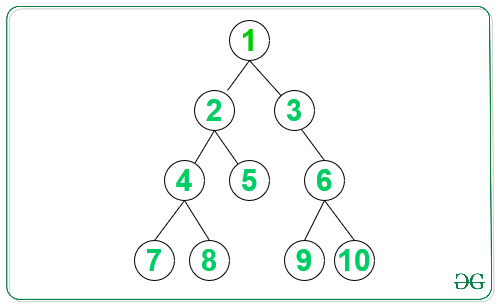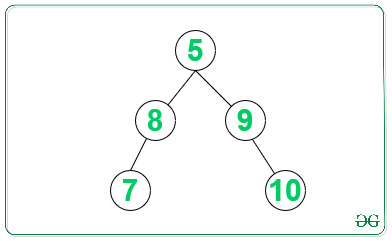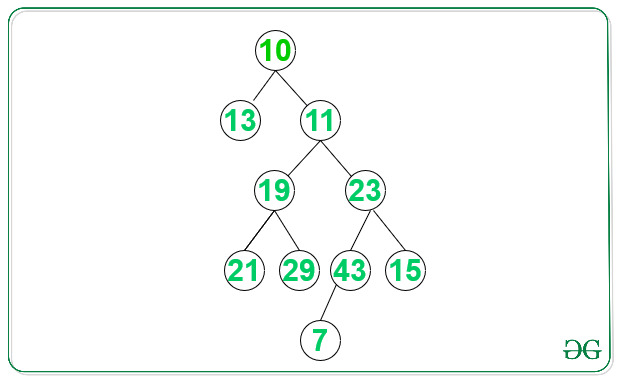# Create Balanced Binary Tree using its Leaf Nodes without using extra space

Prerequisites: Binary Tree to Doubly Linked List

Given a Binary Tree, the task is create a Balanced Binary Tree from all the leaf nodes of the given Binary Tree.

Examples:

```Input:Output: 7 8 5 9 10
Explanation:
Required balanced binary tree will be:Input:Output: 13 21 29 7 15
Explanation:
Required balanced binary tree is:
29
/  \
21   7
/       \
13       15
```

## Recommended: Please try your approach on {IDE} first, before moving on to the solution.

Approach:
Follow the steps below to solve the problem:

1. Find all the leaf nodes in the given binary tree and create a doubly linked list using them.
2. To create Balanced Binary Tree from the above doubly linked list do the following:
• Find the middle node of the doubly linked list formed above and set it as a root node of the resultant tree.
• Recursively iterate for the left and right of the current middle node in the doubly linked list repeat the above steps until all nodes are covered.
3. Print the the newly created balanced binary tree.

Below is the implementation of the above approach:

## C++

 `// C++ program for the above approach ` `#include ` `using` `namespace` `std; ` ` `  `// Structure for Linked list and tree ` `class` `Node { ` `public``: ` `    ``int` `data; ` `    ``Node *left, *right; ` `}; ` ` `  `// Function that returns the count of ` `// nodes in the given linked list ` `int` `countNodes(Node* head) ` `{ ` `    ``// Initialize count ` `    ``int` `count = 0; ` ` `  `    ``Node* temp = head; ` ` `  `    ``// Iterate till the end of LL ` `    ``while` `(temp) { ` ` `  `        ``temp = temp->right; ` ` `  `        ``// Increment the count ` `        ``count++; ` `    ``} ` ` `  `    ``// Return the final count ` `    ``return` `count; ` `} ` ` `  `// Function to return the root of ` `// the newly created balanced binary ` `// tree from the given doubly LL ` `Node* sortedListToBSTRecur( ` `    ``Node** head_ref, ``int` `n) ` `{ ` `    ``// Base Case ` `    ``if` `(n <= 0) ` `        ``return` `NULL; ` ` `  `    ``// Recursively construct ` `    ``// the left subtree ` `    ``Node* left = sortedListToBSTRecur( ` `        ``head_ref, n / 2); ` ` `  `    ``// head_ref now refers to ` `    ``// middle node, make middle node ` `    ``// as root of BST ` `    ``Node* root = *head_ref; ` ` `  `    ``// Set pointer to left subtree ` `    ``root->left = left; ` ` `  `    ``// Change head pointer of ` `    ``// Linked List for parent ` `    ``// recursive calls ` `    ``*head_ref = (*head_ref)->right; ` ` `  `    ``// Recursively construct the ` `    ``// right subtree and link it ` `    ``// with root ` `        ``root->right ` `        ``= sortedListToBSTRecur( ` `            ``head_ref, n - n / 2 - 1); ` ` `  `    ``// Return the root of Balanced BT ` `    ``return` `root; ` `} ` `Node* sortedListToBST(Node* head) ` `{ ` `    ``/*Count the number of  ` `    ``nodes in Linked List */` `    ``int` `n = countNodes(head); ` ` `  `    ``/* Construct BST */` `    ``return` `sortedListToBSTRecur( ` `        ``&head, n); ` `} ` ` `  `// Function to find the leaf nodes and ` `// make the doubly linked list of ` `// those nodes ` `Node* extractLeafList(Node* root, ` `                      ``Node** head_ref) ` `{ ` `    ``// Base cases ` `    ``if` `(root == NULL) ` `        ``return` `NULL; ` ` `  `    ``if` `(root->left == NULL ` `        ``&& root->right == NULL) { ` ` `  `        ``// This node is added to doubly ` `        ``// linked list of leaves, and ` `        ``// set right pointer of this ` `        ``// node as previous head of DLL ` `        ``root->right = *head_ref; ` ` `  `        ``// Change left pointer ` `        ``// of previous head ` `        ``if` `(*head_ref != NULL) ` `            ``(*head_ref)->left = root; ` ` `  `        ``// Change head of linked list ` `        ``*head_ref = root; ` ` `  `        ``// Return new root ` `        ``return` `NULL; ` `    ``} ` ` `  `    ``// Recur for right & left subtrees ` `    ``root->right = extractLeafList(root->right, ` `                                  ``head_ref); ` `    ``root->left = extractLeafList(root->left, ` `                                 ``head_ref); ` ` `  `    ``// Return the root ` `    ``return` `root; ` `} ` ` `  `// Function to allocating new Node ` `// int Binary Tree ` `Node* newNode(``int` `data) ` `{ ` `    ``Node* node = ``new` `Node(); ` `    ``node->data = data; ` `    ``node->left = NULL; ` `    ``node->right = NULL; ` `    ``return` `node; ` `} ` ` `  `// Function for inorder traversal ` `void` `print(Node* root) ` `{ ` `    ``// If root is not NULL ` `    ``if` `(root != NULL) { ` ` `  `        ``print(root->left); ` ` `  `        ``// Print the root's data ` `        ``cout << root->data ` `             ``<< ``" "``; ` `        ``print(root->right); ` `    ``} ` `} ` ` `  `// Function to display nodes of DLL ` `void` `printList(Node* head) ` `{ ` `    ``while` `(head) { ` ` `  `        ``// Print the data ` `        ``cout << head->data << ``" "``; ` `        ``head = head->right; ` `    ``} ` `} ` ` `  `// Driver Code ` `int` `main() ` `{ ` `    ``// Given Binary Tree ` `    ``Node* head = NULL; ` `    ``Node* root = newNode(1); ` ` `  `    ``root->left = newNode(2); ` `    ``root->right = newNode(3); ` `    ``root->left->left = newNode(4); ` `    ``root->left->right = newNode(5); ` `    ``root->right->right = newNode(6); ` `    ``root->left->left->left = newNode(7); ` `    ``root->left->left->right = newNode(8); ` `    ``root->right->right->left = newNode(9); ` `    ``root->right->right->right = newNode(10); ` ` `  `    ``// Function Call to extract leaf Node ` `    ``root = extractLeafList( ` `        ``root, &head); ` ` `  `    ``// Function Call to create Balanced BT ` `    ``root = sortedListToBST(head); ` ` `  `    ``// Print Inorder traversal New Balanced BT ` `    ``print(root); ` `    ``return` `0; ` `} `

Output:

```7 8 5 9 10
```

Time Complexity: O(N), where N is the number of nodes in the given tree.
Auxiliary Space Complexity: O(1)

Attention reader! Don’t stop learning now. Get hold of all the important DSA concepts with the DSA Self Paced Course at a student-friendly price and become industry ready.

My Personal Notes arrow_drop_upCheck out this Author's contributed articles.

If you like GeeksforGeeks and would like to contribute, you can also write an article using contribute.geeksforgeeks.org or mail your article to contribute@geeksforgeeks.org. See your article appearing on the GeeksforGeeks main page and help other Geeks.

Please Improve this article if you find anything incorrect by clicking on the "Improve Article" button below.

Improved By : nidhi_biet